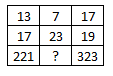Question 16

# Select the missing number from the given responsesSolution

In each column, the last number is obtained by multiplying the other two.

Eg : $$13\times17=221$$

and $$17\times19=323$$

Similarly, $$7\times23=161$$

=> Ans - (D)